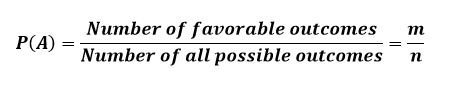Select Page

# Probability Definition

”If an experiment can result in n equally likely, mutually exclusive and exhaustive outcomes and m of which are favorable to the occurrences of an event A, the probability that the event A will occur is given by the ratio m/n. Symbolically, the probability of occurrence of the event A, denoted by P(A), is given by P(A)=m/n.Therefore,## Probability Examples

1. When a fair coin is tossed, what is the probability of getting (i) head (ii) tail?

• When we toss a coin, there are two possible outcomes: head and tail. Since the coin is fair and only one face (head or tail) can come up, the number of equally likely, mutually exclusive and exhaustive outcomes is 2, i.e. n = 2. There is only one outcome favorable to the occurrence of a head, i.e. m = 1. Thus the probability of getting a head is P(head) = 1/2.
• Since there is one outcome favorable to the occurrence of a tail, the probability of getting a tail is P(tail) = 1/2.

2. A perfect cubical die is rolled. What is the probability of getting (i) a ‘5’ (ii) an even number?

• Here the number of total possible outcomes is 6 i.e. 1, 2, 3, 4, 5 and 6 which are equally likely because the die is perfect. Since only one face can come up at a time, they are mutually exclusive. There is only one outcome favorable to the occurrence of a ‘5’. Hence the probability getting a ‘5’ is P(5) = 1/6.
• The number of outcomes favorable to the occurrence of an even number is 3 i.e. 2, 4, 6. Hence the probability of getting an even number is P(even) =3/6 = 1/2.

3. From a well shuffled pack of 52 cards, a card is drawn at random. What is the probability that it is;

• A black card
• An ace
• A jack of clubs
• A pictured card
• A jack or queen of diamonds
• There are 52 playing cards in the pack; hence the number of possible outcomes is 52. Since the pack is well shuffled, we reason that each of these outcomes is equally likely. Since only one card can be drawn at a time, they are mutually exclusive. There are 26 black cards in the pack, so the number outcomes favorable to the occurrence of a ‘black card’ is 26. Hence the probability of getting a black card is P(black card) = 26/52 = 1/2.
• There are only 4 aces in the pack and thus the number of outcomes favorable to occurrence of an ace is 4. Hence the probability of getting an ace is P(ace) = 4/ 52 = 1/13.
• There are 13 cards of spades and thus the number of outcomes favorable to occurrence of spades is 13. Hence the probability of getting a spade is P(spade) = 13/52 = 1/4.
• There is only one jack of clubs and thus the number of outcomes favorable to occurrence of jack of clubs is 1. Hence the probability of getting a jack of clubs is P(jack of clubs) = 1/52.
• There are 12 pictured cards (3 cards in each suit: hearts, spades, clubs and diamonds). The number of outcomes favorable to occurrence of a pictured card is 12. Hence the probability of getting a pictured card is P(pictured card) = 12/52 = 3/13.
• There is only one jack of clubs and one queen of diamonds. The number of outcomes favorable to each of these is 1. Hence, the probability of getting a jack of clubs or queen of diamonds is P(jack of clubs or queen of diamonds) = (1/52) + (1/52) = 1/26.

4. A bag contains 6 red and 4 white balls. Two balls are drawn at random from the bag. Find the probability that (i) both are white (ii) one is red and one is white.

• There are 10 balls in the bag. Two balls out of 10 balls can be drawn in 10C2 = 45 ways.
• Two white balls out of 4 white balls can be drawn in 4C2 = 6 ways. Hence P(two white balls) = 6/45 = 2/15.
• One red ball out of 6 red balls and one white ball out of 4 white balls can be drawn in 6C1 x 4C1 = 6 x 4 = 24 ways. Hence P(one red and one white balls) = 24/45 = 8/15.

5. A box contains 14 identical balls of which 6 are white, 5 red and 3 black. Four balls are drawn. What is the probability that (i) two are red (ii) one is white?

• There are 14 balls in the bag. Four balls out of 14 balls can be drawn in 14C4 = 1001 ways.
• Two red balls out of 5 red balls and two balls out of remaining 9 balls can be drawn in 5C2 x 9C2 = 10 x 36 = 360 ways. Hence P(two red balls) = 360/1001.
• One white ball out of 6 white balls and 3 balls out pf the remaining 8 balls can be drawn in 6C1 x 8C3 = 6 x 56 = 336 ways. Hence P(one white ball) = 336/1001.

To understand probability from all dimension you must go through the following topics:

What is Sample Space, Events and Experiment ?

Dependent and Independent and Conditional Probability

Shares